## General Mechanical

•Maria
Subscriber

Hi,

I am looking for relation between stress and strain in plastic  region.

In multilinear Isotropic hardening I put stress-strain curve, WHERE is the end point ultimate stress, and when ultimate  stress is achieved, ansys keeps the stress on constant value, but strain grows. But which is relation between stress and strain in this region, because stress is const. untile strain grows. In linear region it is the stress = strain x Young module.

•peteroznewman
Subscriber

Hi Maria,

In a physical tensile test, the sample is pulled through the linear range, past the yield point, up to the ultimate strength, then necking occurs followed by fracture at the value of total strain called "elongation at break", a material property.

In a Static Structural model of a tensile test, a multilinear, isotropic hardening, plasticity material model may contain the data (converted to true stress and plastic strain) up to the ultimate stress, then the stress is constant for higher values of strain.

A displacement controlled model can be pulled through the linear range, past the yield point and the stress can get to the ultimate stress, but the elements will continue to stretch and plastic strain will continue to increase way past the "elongation at break" value of total strain. In other words, you will not see a fracture in the model. The elements will continue to stretch until the element shape has become so distorted that calculation of results becomes invalid.

You, as the engineering analyst, will predict that in this non-linear analysis, the part has fractured when you see the Total Strain result has exceeded the "elongation" value.  This is similar to you being able to predict in a linear analysis that the part has yielded when you see the Equivalent Stress has exceeded the yield strength value.

Regards,
Peter

•Sandeep Medikonda
Ansys Employee

Maria,

~Sandeep

•Maria
Subscriber

Peter and Sandeep thank for the response.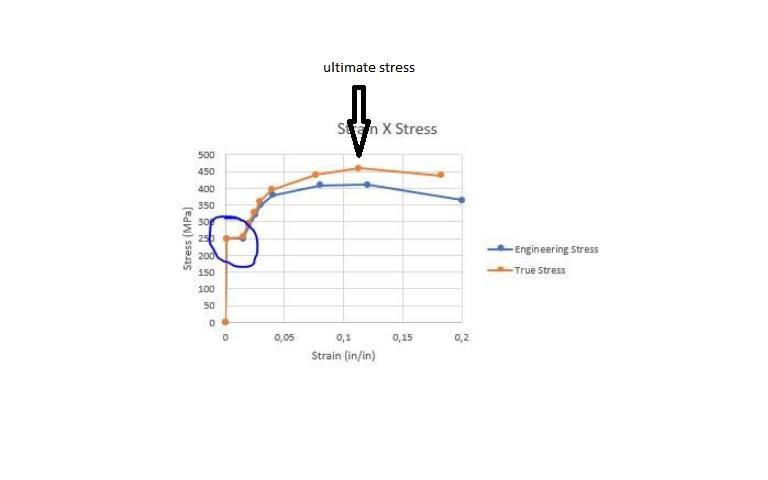I  have seen this pic. in the post which is Sandeep recommend, I am interested about relation between stress and strain. Have anyone example how ansys calculate stress and strain and which is relation between them in fem (elastic, plastic, necking).

After ultimate stress, in the above picture we can see that stress after ultimate stress decrease and because that I am confuse how the ansys assume the stress is constant but strain grows.

For example, we have two beam which are pulled (tensile test), one of them has 2 mm weight and high other has 3mm w and h. I pull them equal force of 300N, and both have equal stress (achieved ultimate stress, as in picture above about 450 MPa) but strain is not equal 2% and 4%. These values is only example no the real value, when we will calculate beams with this weight and higt. But I am looking for response how ansys then calculate strain if stress is const. which is it equation which is used.

Thanks.

•peteroznewman
Subscriber

Maria,

The graph you copied and used above has errors in it.  True Stress vs. True Strain does not have a negative slope, and ANSYS will not allow that.

I am looking for response how ansys then calculate strain if stress is const. which is it equation which is used.

ANSYS assembles a stiffness matrix [K] and solves the matrix equation [K]{x} = {F} for the unknown nodal displacements {x} and the known applied force {F}.

Element strain is computed from nodal displacements.

Element stress is computed from element strain using the material constitutive model.

Since it is a nonlinear system, ANSYS computes the force imbalance, revises the stiffness matrix and solves the equations again to update the nodal displacements until the force imbalance falls below a convergence criteria.

In the link to the discussion with Fabricio, there are some useful articles and videos on plasticity.

•Sandeep Medikonda
Ansys Employee

Maria,

At the ultimate strength the slope of engineering Stress-Strain curve is 0, but, the slope of true Stress-Strain curve is always increasing and positive. This is very important in numerical methods, as it would introduce instabilities into the model. Note that we are inverting the stiffness matrix to calculate the nodal displacements. The simplified relation that was presented in that discussion is only valid for incompressible plasticity stress-strain data (untill necking). Now, Beyond necking, There is no conversion equation relating engineering to true stress-strain at necking. The instantaneous cross-section must be measured.

With regards to your question on different cross-sections, I don't quite follow but maybe this paper could help.

~Sandeep

•Maria
Subscriber

Thanks..

Peter, in last post:

ANSYS assembles a stiffness matrix [K] and solves the matrix equation [K]{x} = {F} for the unknown nodal displacements {x} and the known applied force {F}.

Element strain is computed from nodal displacements.

Please correct me If I am wrong.From the above answer it means that strain does not depend of the stress, it depends of nodal displacements, which can be calculate  by matrix equation, where are known F (force)  and stiffness matrix (Young module).

But I have not understood  what mean about calculation of stress:

Element stress is computed from element strain using the material constitutive model.

Does this mean that stress depends of  construction:    Stress=Applied force(N)/ Surface(m2).  If is tensile test.

You said that the fracture criteria is total strain, if total strain overcomes "elongation at break" on the construction we will predict fracture. The stress what is with him, whether the stress need to reach ultimate stress or can be smaller than ultimate stress. When I input the material properties  the last point is ultimate vs plastic strain (total  - elastic strain, but in this pint total is equal elongation strain at break). Can ansys reach total strain at break but does not reach ultimate stress. If can, whether it because stress does not depend of strain.

Sandeep thanks, I will read this paper.

•Maria
Subscriber

In elongation strain, at the point of maximum stress on the construction we will predict crack but when total strain is about 0,18 % (the above picture)  we predict the construction will break. When I need determine the max force on the  construction, whether it force be at the total strain at crack or at total strain at break.   Because the crack will occur at total strain (elongation strain at break) but the construction does not break. I suppose it is at total stain of break, and we can assume that the construction is good if total strain is less than the total strain at crack (see picture)

Correct me if I am wrong.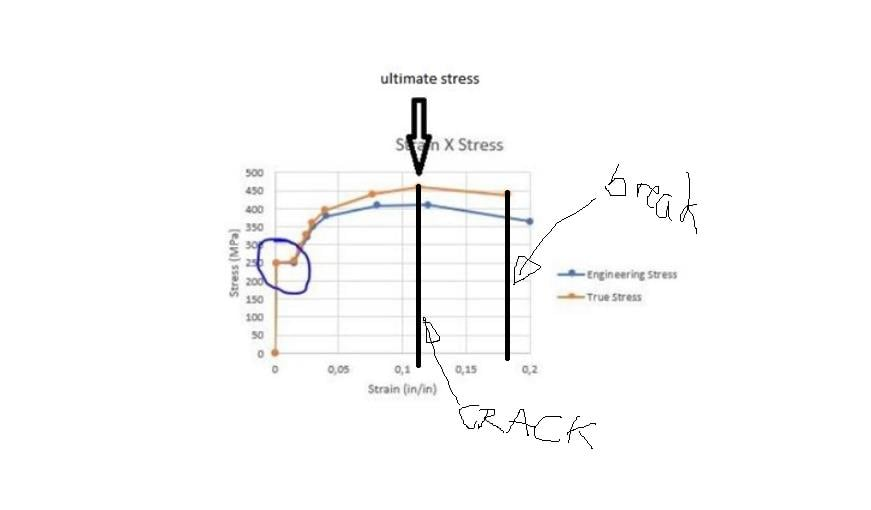Thanks.

•Sandeep Medikonda
Ansys Employee

Maria,

In numerical codes, We solve the equations of motion, where we are iteratively solving for displacements, based on which we are calculating the strain and using the constitutive relations calculating the stresses.

Resource 1

Resource 2

We are not talking about cracks here and its only material yielding in plasticity laws. If your model experiences a strain larger than what you have input, say for multilinear plasticity it takes the last point and just keeps if flat, i.e. it will keep yielding until about 20-30% strain and will eventually start running into convergence troubles. For what you are talking with respect to damage, softening and failure, please refer to Chapter 9 in Resource 2 above.

P.S: Please ignore that plot, it is incorrect.

~Sandeep

•peteroznewman
Subscriber

Maria,

But I have not understood  what mean about calculation of stress:

Element stress is computed from element strain using the material constitutive model.

Does this mean that stress depends of  construction:    Stress=Applied force(N)/ Surface(m2).  If is tensile test.

No, the stress in the numerical computation has nothing to do with applied force, which was already used in step one to solve for the nodal displacements.

Once the element strain is computed from the nodal displacement, a simple lookup on the stress-strain curve computes the stress.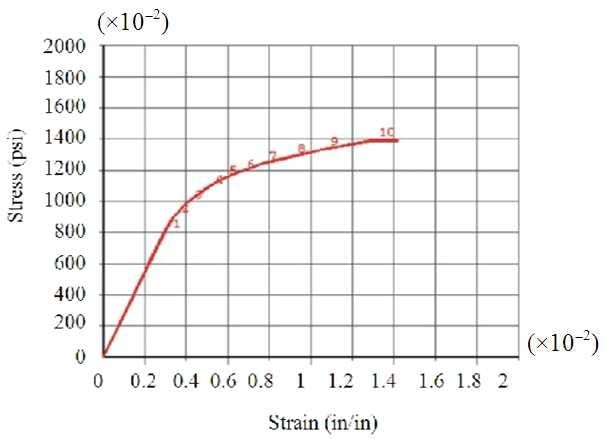Regards,

Peter

•Maria
Subscriber

Peter thanks,

It means that from the above curve stress-strain, if is strain 1,4 in/in, then the stress is about 1400 psi.  Thanks.

I did one example which is attached, simple  beam. Please can me help that it calculate hand and it apply on Ansys.

I do not know how I can know that the results are correct, without hand calculation. I do not know how I can know  that is mesh ok when is the construction is complex. Can I use the curve stress-strain as verification fem analysis. i.e that in the strain 1,4 must be stress about 1400 psi.

Thank one more.

•peteroznewman
Subscriber

Maria,

The problem with the Fixed Support, is it doesn't let the face contract with the Poisson's Ratio of the material and so creates lateral stress in the face that is not considered in a hand calculation.

The way to get an ANSYS tensile model to have the stress you can calculate from Force/area is to use three displacements on three faces, one for X=0 on the face normal to X, a second for Y=0 on the face normal to Y and a third for Z=0 on the face normal to Z. This represents a 1/8 model of the center of a rectangular bar in tension.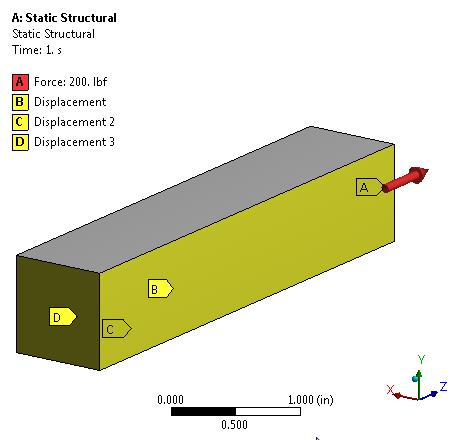Then the stress is uniform in the bar. Here is the Normal Stress in the Z direction.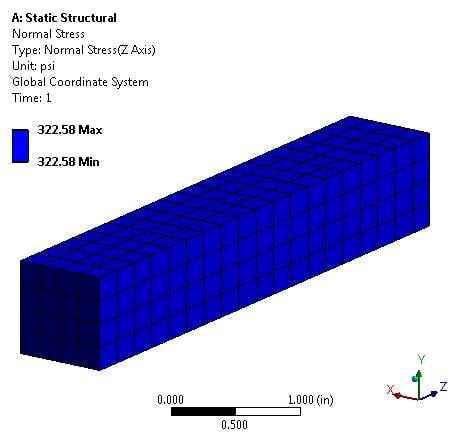The force was 200 lbs, the area is 0.62 sq. in so the stress by hand calculation should be 200/0.62 = 322.58 psi, which is what ANSYS calculated.

Your material model is multilinear. The first stress in the table is the yield stress of 896. This means the result above is in the elastic range.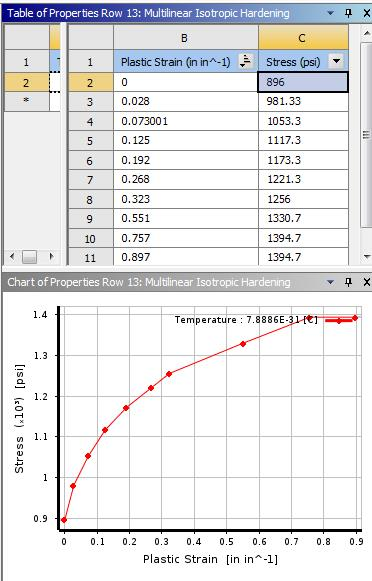In the linear range, the strain depends only on the Young's Modulus of the material. You used E = 2667 psi, so by hand calculation, we expect a strain of Stress/Modulus = 0.12095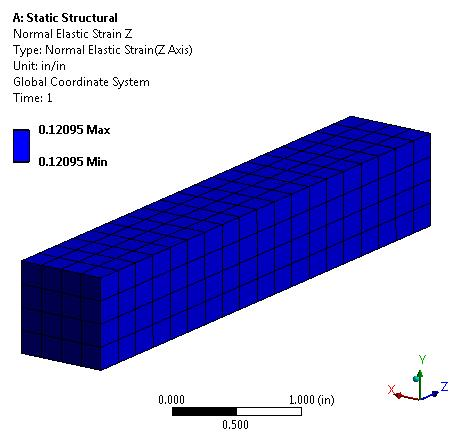If you increase the force, you can get this material into the plastic range.

I hope this helped your understanding.

Regards,

Peter

Attached is an ANSYS 19.1 archive. (I can't easily tell if you use a different version, but you can say what version when you next attach).